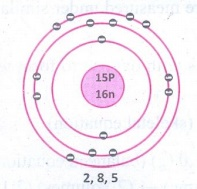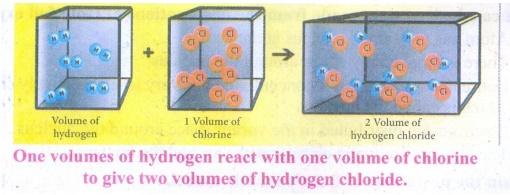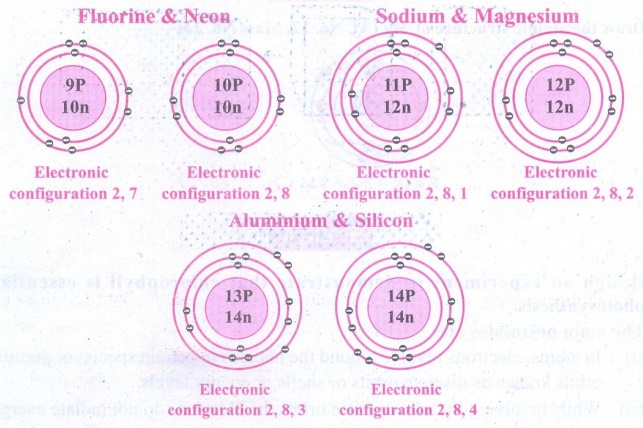Home | | Science 9th std | Answer the following questions

## Chapter: 9th Science : Chemistry : Atomic Structure

Science : Chemistry : Atomic Structure : Book Back Important Questions, Answers, Solutions : Answer briefly, Answer in detail, Exercise Numerical Problems, Intext Activities

CHEMISTRY

ATOMIC STRUCTURE

### TEXT BOOK EXERCISES

1. Name an element which has the same number of electrons in its first and second shell.

Answer: Beryllium. Atomic number - 4 (K-shell-2, L-shell-2)

2. Write the electronic configuration of K and CI3. Write down the names of the particles represented by the following symbols and explain the meaning of superscript and subscript numbers attached. 1H1, 0n1, -1e0

1H1 : hydrogen atom mass number 1. Atom number 1.

0n1 : neutron change 0. Mass 1 amu.

-1e0 : electron change –1. Mass negligible.

4. For an atom ‘X’, K, L and M shells are completely filled. How many electrons will be present in it?

28 [K=2, L=8, M=18]

5. What is the same about the electron structures of:

a. Lithium, Sodium and Potassium.

b. Beryllium, Magnesium and Calcium.

a. Lithium, sodium & potassium have 1 electron in their outermost shell.

b. Beryllium, magnesium and calcium have 2 electrons in their outermost shell.

1. How was it shown that atom has empty space?

Answer: Rutherford performed an experiment of bombarding a thin gold foil with very small positively charged particles called α particles. He observed that most of the alpha particles passed straight through the foil. He inferred by thin experiment that most of the space in the atom is empty.

2. Why do 3517Cl and 3717Cl have the same chemical properties? In what respect do these atoms differ?

Answer:  3517Cl, 3717Cl have same chemical properties because they have same number of electrons but their atoms differ in the number of neutrons.

3. Draw the structure of oxygen and sulphur atoms.4. Calculate the number of neutrons, protons and electrons :

(i) atomic number 3 and mass number 7

(ii) atomic number 92 and mass number 238.

(i) atomic number 3 and mass number 7

∴ No. of electrons (or) No. of protons = 3

Mass number = No. of protons + No. of neutrons

∴ No. of neutrons = 7 – 3 = 4

(ii) atomic number 92 and mass number 238

Atomic number = 92

∴No. of electrons = 92

No. of protons = 92

∴No. of neutrons = 238 − 92 = 146

5. What are nucleons? How many nucleons are present in Phosphorous? Draw its structure.The number of protons and neutrons present in the nucleus are called nucleons. The number of nucleons present is phosphorus is 31.

1. What conclusions were made from the observations of Gold foil experiment?

(i) Atom has very small nucleus at the centre.

(ii) There is large empty space around the nucleus.

(iii) Entire mass of an atom is concentrated in a very small positively charged region which is called the nucleus.

(iv) Electrons are distributed in the vacant space around the nucleus.

(v) The electrons move in circular paths around the nucleus.

2. Explain the postulates of Bohr’s atomic model.

The main postulates are:

(i) In atoms, the electron revolve around the nucleus in stationary circular paths called orbits or shells or energy levels.

(ii) While revolving around the nucleus in an orbit, an electron neither loses nor gains energy.

(iii) An electron in a shell can move to a higher or lower energy shell by absorbing or releasing a fixed amount of energy.

(iv) The orbits or shells are represented by the letters K,L,M,N,... or the numbers, n = 1,2,3,4,....Energy levels around the nucleus of an atom : Bohr’s model.

The orbit closest to the nucleus is the K shell. It has the least amount of energy and the electrons present in it are called K electrons, and so on with the successive shells and their electrons. These orbits, are associated with fixed amount of energy, so Bohr called them as energy level or energy shells.

3. State the Gay Lussac’s law of combining volumes, explain with an illustration.

Whenever gases react together, the volumes of the reacting gases bear a simple ratio, and the ratio is extended to the product when the product is also in gaseous state, provided all the volumes are measured under similar conditions of temperature and pressure.

Example:

Step 1: Hydrogen combines with oxygen to form water (word equation) Hydrogen + Oxygen → Water.

Step 2: H2 + (1/2) O2 → H2O (skeletal equation)

Step 3: 2H2(g) + O2(g) → 2H2O (g) (balanced equation)

(2 Volumes) + (1 Volume) → (2 Volumes) (2:1:2)

This law may be illustrated by the following example.

It has been experimentally observed that two volumes of hydrogen reacts with one volume of oxygen to form two volumes of water.

The ratio of volume which gases bears is 2:1:2 which is a simple whole number ratio.Intext Activities

ACTIVITY - 1

Symbolically represent the following atoms using atomic number and mass number.

a) Carbon

b) Oxygen

c) Silicon

d) Beryllium

a) Carbon – 126C

b) Oxygen – 168O

c) Silicon – 2814Si

d) Beryllium – 94Be

ACTIVITY - 2

Assign the valency for Phosphorus, Chlorine, Silicon and Argon

Phosphorus - P – 3.5

Chlorine – Cl - 1

Silicon - Si - 4

Argon - Ar - 0

ACTIVITY - 4

Draw the structures of the isotopes of oxygen O16 and O18. Atomic number of oxygen = 8.ACTIVITY - 5

Draw the model of the following pairs of isotones:

(i) Fluorine & Neon

(ii) Sodium & Magnesium

(iii) Aluminum and Silicon.ACTIVITY - 6

Nitrogen combines with hydrogen to form ammonia (NH3). Illustrate Gay Lussac’s law using this example.

Step 1: Nitrogen ↑ + Hydrogen ↑ → Ammonia ↑.

Step 2: N2 ↑ + H2 ↑ → NH3 ↑.

Step 2: N2 ↑ + 3H2 2NH3 ↑.

(1 volume ) + (3 volume ) (2 volume )

1 volume of nitrogen reacts with 3 volumes of hydrogen to form 2 volumes of ammonia gas. The ratio by volume which gases bear is 1 : 3 : 2.Which is a simple while number ratio.

Test Yourself:

1. Calculate the number of neutrons in the following atoms:

a) 2713Al

b) 3415P

c) 19076Os

d) 5424Cr

a) 2713Al ⇒ Number of neutrons (n) = 27 – 13 = 14

b) 3415P ⇒ Number of neutrons (n) = 31 – 15 = 16

c) 19076Os ⇒ Number of neutrons (n) = 190 – 76 = 114

d) 5424Cr ⇒ Number of neutrons (n) = 54 – 24 = 30

Tags : Atomic Structure | Chemistry | Science , 9th Science : Chemistry : Atomic Structure
Study Material, Lecturing Notes, Assignment, Reference, Wiki description explanation, brief detail
9th Science : Chemistry : Atomic Structure : Answer the following questions | Atomic Structure | Chemistry | Science